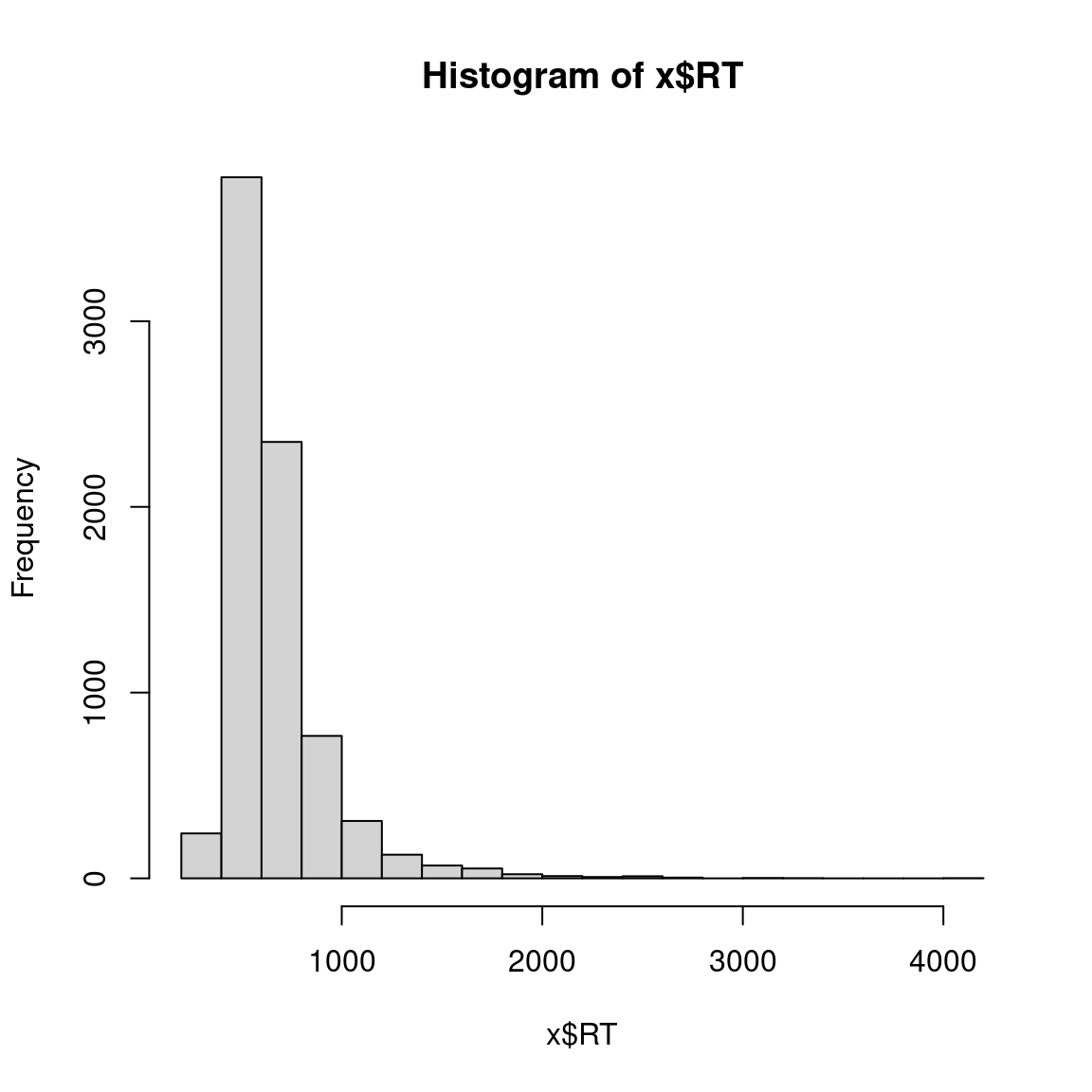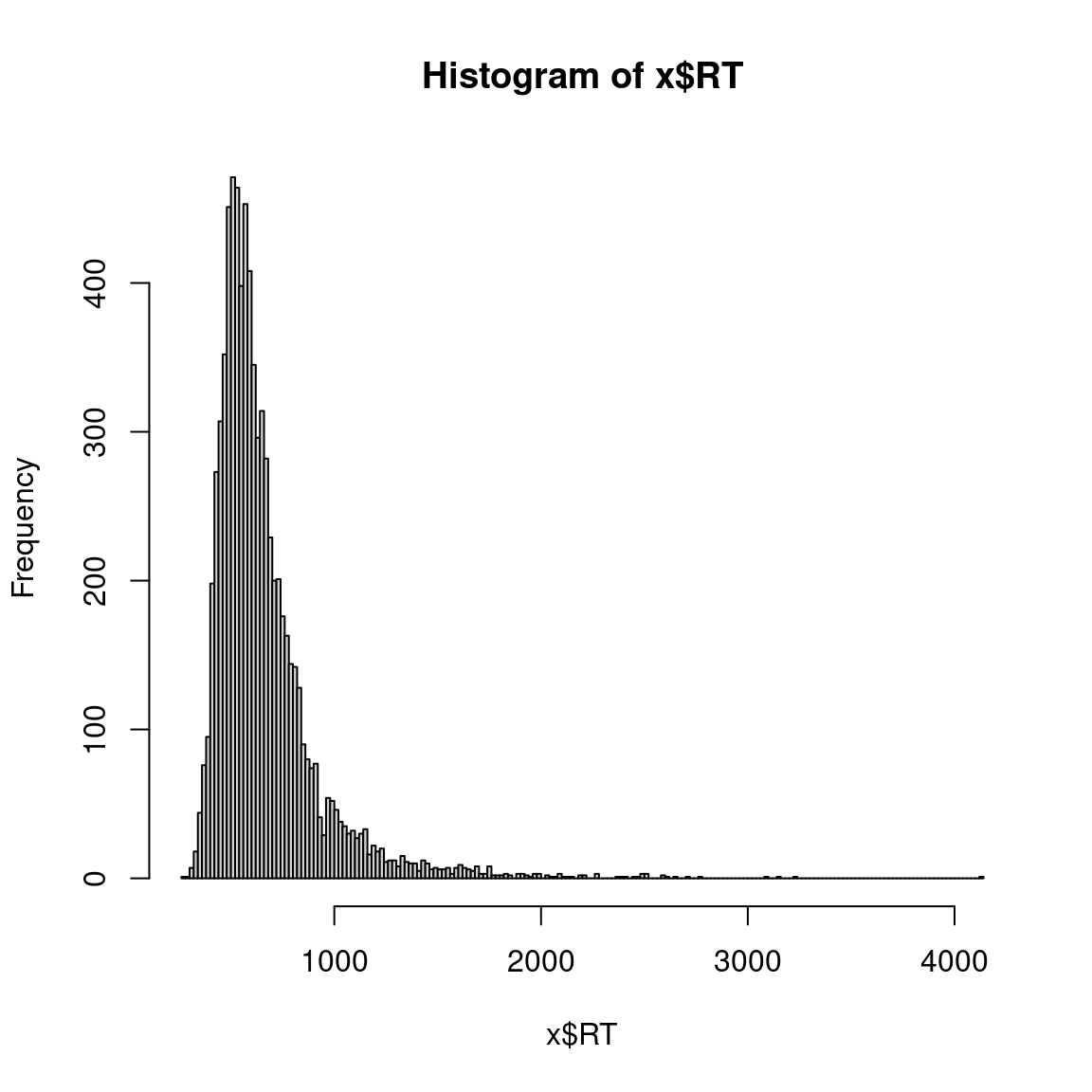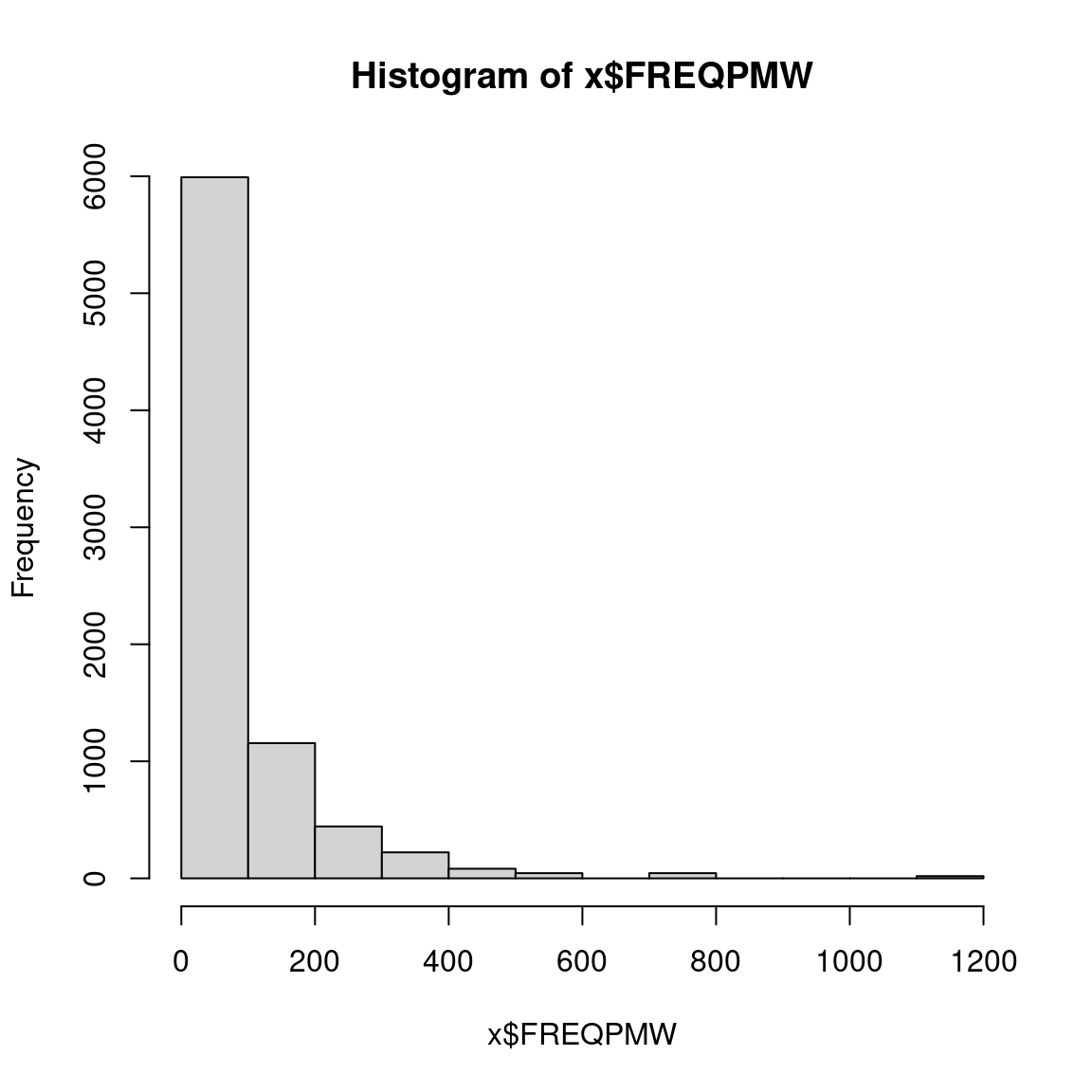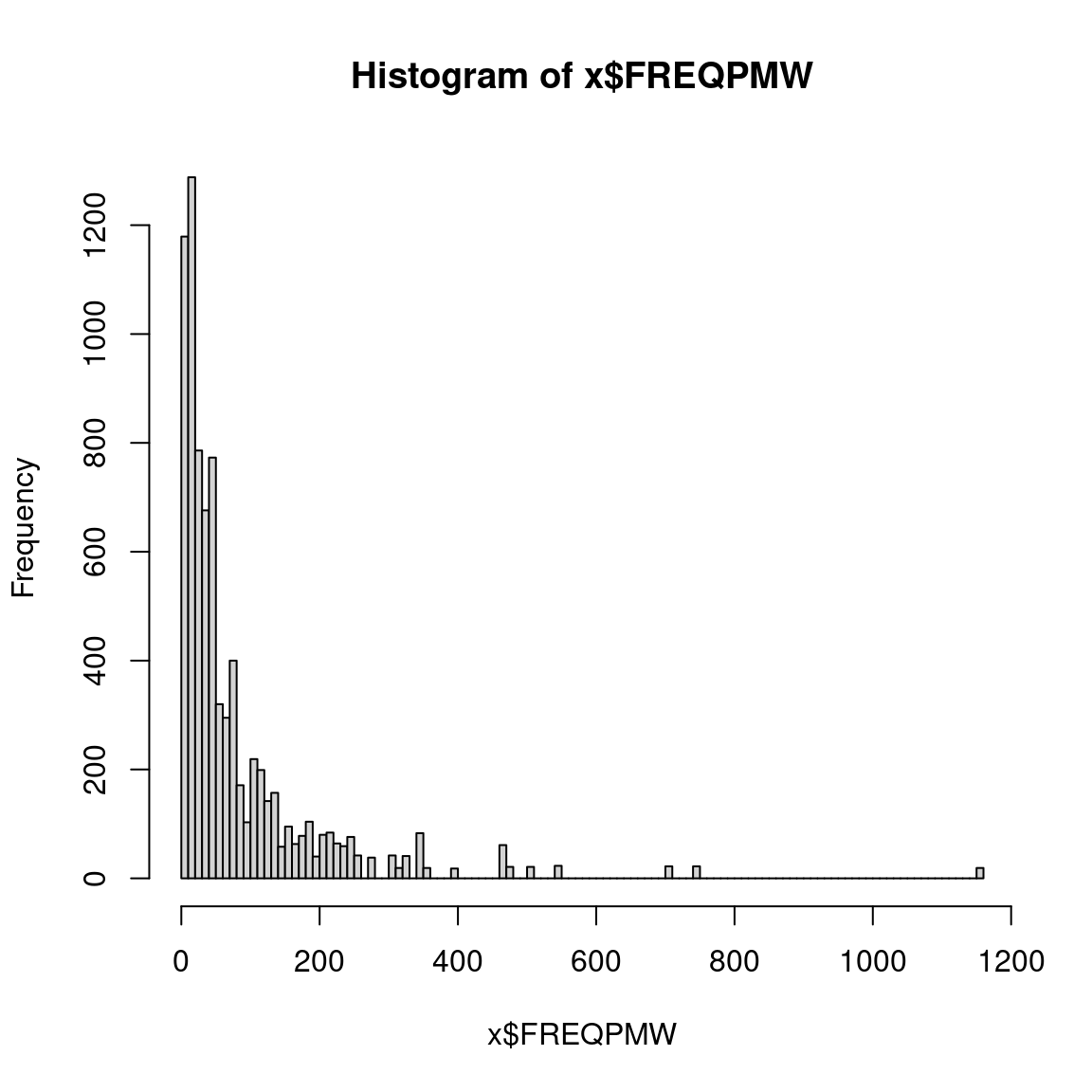# 1 Session 02: Linear modeling 1

## 1.1 Class activities

``````rm(list=ls(all.names=TRUE))
library(car); library(effects)
file="105_02-03_RTs.csv", # this file
stringsAsFactors=TRUE))   # change categorical variables into factors``````
``````##       CASE            RT             LENGTH      LANGUAGE        GROUP
##  Min.   :   1   Min.   : 271.0   Min.   :3.000   eng:4023   english :3961
##  1st Qu.:2150   1st Qu.: 505.0   1st Qu.:4.000   spa:3977   heritage:4039
##  Median :4310   Median : 595.0   Median :5.000
##  Mean   :4303   Mean   : 661.5   Mean   :5.198
##  3rd Qu.:6450   3rd Qu.: 732.0   3rd Qu.:6.000
##  Max.   :8610   Max.   :4130.0   Max.   :9.000
##                 NA's   :248
##       CORRECT        FREQPMW           ZIPFFREQ     SITE
##  correct  :7749   Min.   :   1.00   Min.   :3.000   a:2403
##  incorrect: 251   1st Qu.:  17.00   1st Qu.:4.230   b:2815
##                   Median :  42.00   Median :4.623   c:2782
##                   Mean   :  81.14   Mean   :4.591
##                   3rd Qu.: 101.00   3rd Qu.:5.004
##                   Max.   :1152.00   Max.   :6.061
## ``````

### 1.1.1 Deviance

A useful thing to consider is deviance, which we will here define as the overall amount of variability in the response variable. As wet talked about before in class, the response variable has a mean, but the response variable’s values are not all the mean, they vary around it:

``summary(x\$RT)``
``````##    Min. 1st Qu.  Median    Mean 3rd Qu.    Max.    NA's
##   271.0   505.0   595.0   661.5   732.0  4130.0     248``````

One way to express the degree to which the values of `RT` vary around the mean would be the average absolute deviation:

``mean(abs(x\$RT - mean(x\$RT, na.rm=TRUE)), na.rm=TRUE)``
``##  172.5416``

But as you know, this is not how we usually quantify deviations from the mean: as in the standard deviation, we usually square the differences between the observed values and the mean and then add them up to get the deviance:

``sum((x\$RT - mean(x\$RT, na.rm=TRUE))^2, na.rm=TRUE)``
``##  536471586``

This number quantifies how much all data points together deviate from the mean. Another useful way to look at this would be to already use a linear model quantify this variability of the values around the mean. We can do this by fitting a so-called null model, i.e. a model with no predictors:

``````summary(m.00 <- lm(       # make/summarize the linear model m.01:
RT ~ 1,                # RT ~ an overall intercept (1) & no predictor
data=x,                # those variables are in x
na.action=na.exclude)) # skip cases with NA/missing data (good habit)``````
``````##
## Call:
## lm(formula = RT ~ 1, data = x, na.action = na.exclude)
##
## Residuals:
##    Min     1Q Median     3Q    Max
## -390.5 -156.5  -66.5   70.5 3468.5
##
## Coefficients:
##             Estimate Std. Error t value Pr(>|t|)
## (Intercept)  661.469      2.988   221.4   <2e-16 ***
## ---
## Signif. codes:  0 '***' 0.001 '**' 0.01 '*' 0.05 '.' 0.1 ' ' 1
##
## Residual standard error: 263.1 on 7751 degrees of freedom
##   (248 observations deleted due to missingness)``````

That model’s deviance is the same as what we computed manually just above:

``deviance(m.00)``
``##  536471586``

Note that this null model actually returns the same result as when you use a one-sample t-test on the reaction times:

``t.test(x\$RT)``
``````##
##  One Sample t-test
##
## data:  x\$RT
## t = 221.37, df = 7751, p-value < 2.2e-16
## alternative hypothesis: true mean is not equal to 0
## 95 percent confidence interval:
##  655.6111 667.3259
## sample estimates:
## mean of x
##  661.4685``````

And this is how we’d get the confidence intervals for the intercept or coefficients more generally:

``Confint(m.00)``
``````##             Estimate    2.5 %   97.5 %
## (Intercept) 661.4685 655.6111 667.3259``````

And the manual computation would be this:

``````# lower bound:
coef(m.00) +              # the intercept plus
summary(m.00)\$coef[1,2] * # the intercept's se
qt(0.025, df=7751)        # the t-value for 2.5% at that df``````
``````## (Intercept)
##    655.6111``````
``````# upper bound:
coef(m.00) +              # the intercept plus
summary(m.00)\$coef[1,2] * # the intercept's se
qt(0.975, df=7751)        # the t-value for 2.5% at that df``````
``````## (Intercept)
##    667.3259``````

Since our above deviance value is the overall amount of variability of the response variable and, at the same time, an expression of how bad a model with no predictors is at making predictions, we are now of course hoping that our predictors can reduce that number …

### 1.1.2 A numeric predictor

Does the reaction time to a word (in ms) vary as a function of the frequency of that word (normalized to per million words)?

Some exploration of the relevant variables:

``````# the predictor(s)/response on its/their own
hist(x\$RT); hist(x\$RT, breaks="FD")``````Very long tail on the right … Let’s see where ‘the middle 90%’ of the data are, which might be useful as a good plotting range:

``quantile(x\$RT, probs=seq(0, 1, 0.05), na.rm=TRUE)``
``````##      0%      5%     10%     15%     20%     25%     30%     35%     40%     45%
##  271.00  417.00  446.00  469.65  487.00  505.00  522.00  538.00  556.00  576.00
##     50%     55%     60%     65%     70%     75%     80%     85%     90%     95%
##  595.00  614.00  640.00  665.00  695.00  732.00  777.00  834.00  929.00 1137.45
##    100%
## 4130.00``````

Ok, so the middle 90% of the data are in [417, 1137.45], which means that something like `c(400, 1150)` might make for good y-axis limits (at least for regression predictions). Now the predictor:

``hist(x\$FREQPMW); hist(x\$FREQPMW, breaks="FD")````````# the predictor(s) w/ the response
plot(
main="RT in ms as a function of frequency per million words",
pch=16, col="#00000030",
xlab="Frequency pmw", xlim=c(0, 1200)  , x=x\$FREQPMW,
ylab="RT in ms"     , ylim=c(250, 4250), y=x\$RT); grid()
abline(lm(x\$RT ~ x\$FREQPMW), col="red", lwd=2)``````